: 2 Atmospheric model : Two dimensional anelastic model : \$BL\

# 1 Outline of discretizatoin

The outline of finite difference method adapted for this model is as follows.

Space differencing
The finite difference form of governing equations of the model are considered on the Lorenz type staggered grid. The space differencing is evaluated by the forth order centered scheme for scalar advection terms and the continuity equation, and the second order centered scheme for others. The numerical diffusion is introduced to the equation of motion, turbulent kinetic energy equation, and advection diffusion equation of dust so that the 2-grid noise associated with central finite differencing can be suppressed. The numerical diffusion in equation of motion is proportional to the squared wind shear and that in turbulent kinetic energy equation, and advection diffusion equation of dust is proportional to the third power of Laplace operator.

The vertical integral in calculating CO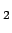infrared radiative flux is evaluated by the trapezoidal rule.

Time differencing
The time integration is performed by the leap-frog scheme for advection and buoyancy terms and the forward scheme for turbulent diffusion and forcing terms. The forward scheme is also adapted once per 20 steps for advection and buoyancy terms to stabilized numerical solution. The radiative flux associated with dust is given by iteration method of the matrix equation, where the number of iteration is 4. The time integration of 1D thermal conduction equation of grand surface is performed by the Crank-Nicolson scheme.

In the following sections, the subscripts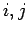show horizontal and vertical grid point, and the superscripts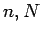show time step. The number of vertical grid level is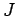. The scalar and basic state variables are evaluated on the grid point and the other variables are evaluated on the half grid point.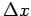and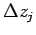are the horizontal and vertical grid intervals, and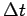is the time interval.: 2 Atmospheric model : Two dimensional anelastic model : \$BL\
Odaka Masatsugu \$BJ?@.(B19\$BG/(B4\$B7n(B26\$BF|(B